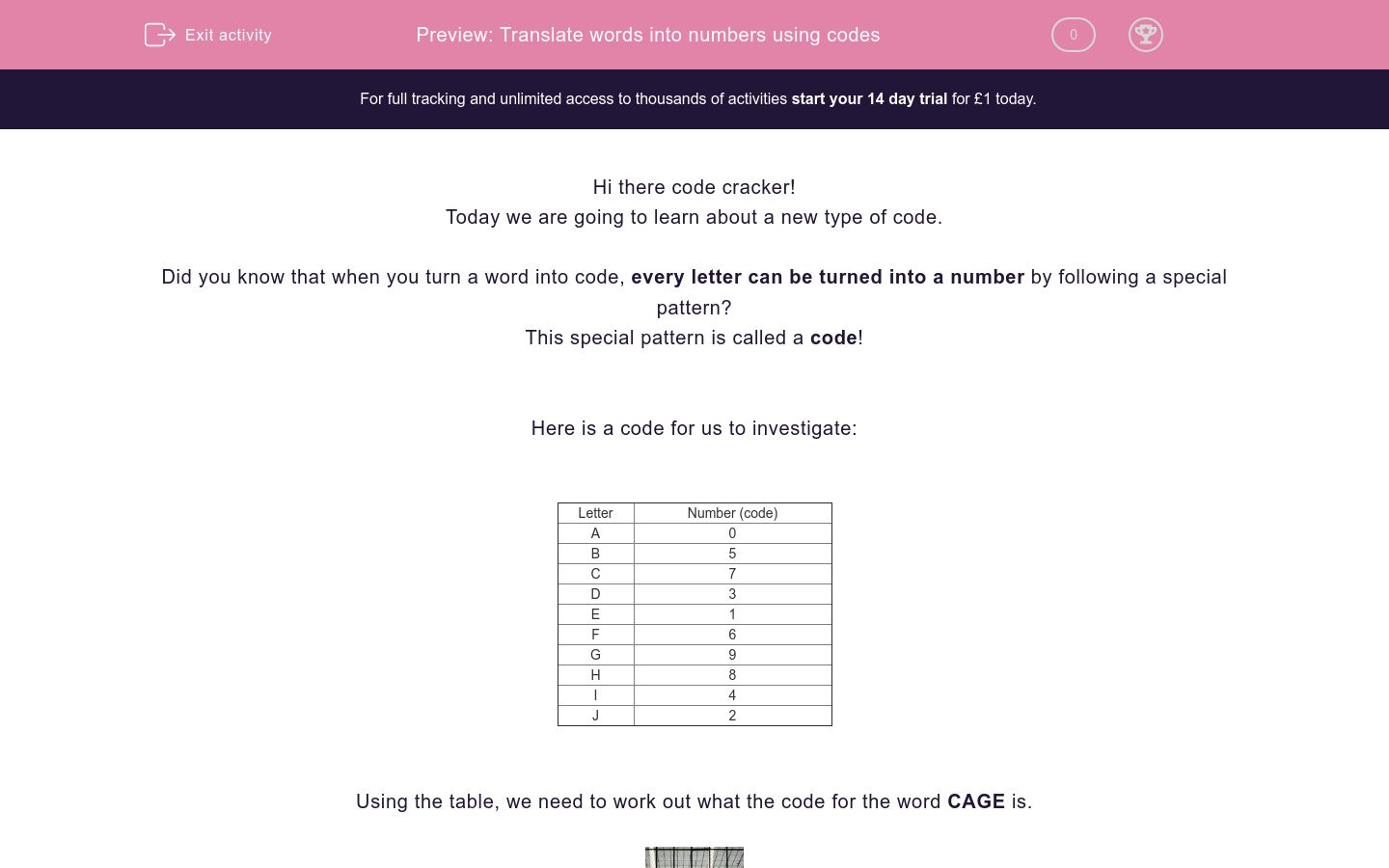# Translate words into numbers using codes

In this worksheet, students will turn words into number codes using a given code table as a base. It will develop their encoding and problem solving skills.Key stage:  KS 2

Curriculum topic:   Verbal Reasoning

Curriculum subtopic:   Number Code Breaking

Difficulty level:### QUESTION 1 of 10

Hi there code cracker!

Today we are going to learn about a new type of code.

Did you know that when you turn a word into code, every letter can be turned into a number by following a special pattern?

This special pattern is called a code!

Here is a code for us to investigate:

 Letter Number (code) A 0 B 5 C 7 D 3 E 1 F 6 G 9 H 8 I 4 J 2

Using the table, we need to work out what the code for the word CAGE is.If we start with finding the code for C and then work through each letter one-by-one, we find that the code for CAGE is 7091.

Now let’s reverse what we did to find out which word is represented by the code 8103.

Then we can repeat this process for each of the numbers one-by-one to find that the word is HEAD.

Is that what you found?

It’s now your turn to be a code cracker and change words into and out of code. Make sure you check the code table for each question.

Good luck code cracker!

---- OR ----